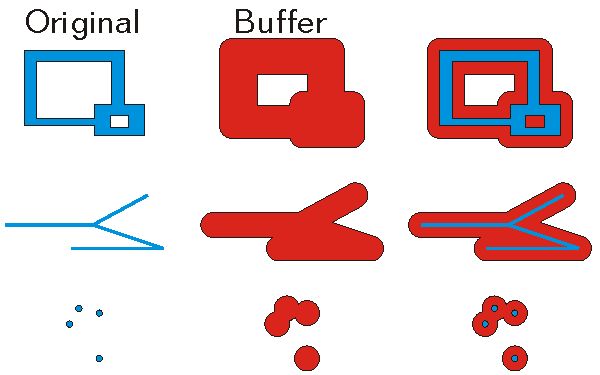This document is archived and information here might be outdated.  Recommended version.

ITopologicalOperator.Buffer Method (ArcObjects .NET 10.7 SDK)
 ArcObjects Help for .NET developers > ArcObjects Help for .NET developers > ArcObjects namespaces > Geometry > ESRI.ArcGIS.Geometry > Interfaces > IT > ITopologicalOperator Interface > ITopologicalOperator.Buffer Method
 ArcGIS Developer Help

# ITopologicalOperator.Buffer Method

Constructs a polygon that is the locus of points at a distance less than or equal to a specified distance from this geometry.

```[Visual Basic .NET]
Public Function Buffer ( _
ByVal distance As Double _
) As IGeometry
```
```[C#]
public IGeometry Buffer (
double distance
);
```
```[C++]
HRESULT Buffer(
double distance
);
```
```[C++]
Parameters
distance
distance is a parameter of type double
```

#### Product Availability

Available with ArcGIS Engine, ArcGIS Desktop, and ArcGIS Server.

#### Remarks

The buffer distance is in the same units as the source shape that is being buffered.

A negative distance can be specified to produce a buffer inside the original polygon. This cannot be used with polyline.

ITopologicalOperator methods must be applied on top-level geometries only. Top-Level geometries are point, multipoint, polyline and polygon. To use this method with segments (Line, Circular Arc, Elliptic Arc, B�zier Curve), paths or rings, they must be wrapped with a top-level type.

A buffer distance of 0 will generate an empty polygon with the input geometry being a polyline and multipoint.  However, if the input geometry is a point, the output will be the original point.[C#]

//The following code shows to wrap a line segment into a polyline in C#

//Assume a line (line1 as ILine) is already created

object obj = Type.Missing;

ISegmentCollection segCollection = new PolylineClass() as ISegmentCollection;

segCollection.AddSegment((ISegment)line1, ref obj, ref obj);

//Set the spatial reference on the new polyline

//The spatial reference is not transfered automatically from the segments

IGeometry geom = segCollection as IGeometry;

geom.SpatialReference = spatialRef;

//Can now be used with ITopologicalOperator methods

[C#]//This example demonstrates how to use ITopologicalOperator::Buffer
private void BufferArea()
{
IPoint[] points = new IPoint;
//The spatial reference should be set here using IGeometry::SpatialReference (Code skipped here)
for(int i = 0; i &lt; points.Length; i++)
{
points[i] = new PointClass();
}
points.PutCoords(0, 0);
points.PutCoords(0, 10);
points.PutCoords(10, 10);
points.PutCoords(10, 0);
points.PutCoords(0, 0);
IPointCollection4 pointCollection = new PolygonClass();
IGeometryBridge geometryBride = new GeometryEnvironmentClass();
IArea area = pointCollection as IArea;
System.Windows.Forms.MessageBox.Show("Area original polygon : " + area.Area);
ITopologicalOperator topologicalOperator = pointCollection as ITopologicalOperator;
//Outside buffer
IPolygon polygon = topologicalOperator.Buffer(1) as IPolygon;
area = polygon as IArea;
System.Windows.Forms.MessageBox.Show( "Area polygon positive distance : " + area.Area);
//Inside buffer
polygon = topologicalOperator.Buffer(-1) as IPolygon;
area = polygon as IArea;
System.Windows.Forms.MessageBox.Show("Area polygon negative distance : " + area.Area);
}

[C++]
[Visual Basic .NET]

Sub exampleITopologicalOperator_Buffer()
Dim ptc As ESRI.ArcGIS.Geometry.IPointCollection, i As Long, pa As ESRI.ArcGIS.Geometry.IArea, ptopo As ESRI.ArcGIS.Geometry.ITopologicalOperator
ptc = New ESRI.ArcGIS.Geometry.Polygon
Dim pt(4) As ESRI.ArcGIS.Geometry.IPoint, poutPoly As ESRI.ArcGIS.Geometry.IPolygon
'The spatial reference should be set here using IGeometry::SpatialReference (Code skipped here)
For i = 0 To 4
pt(i) = New ESRI.ArcGIS.Geometry.Point
Next
pt(0).PutCoords(0, 0)
pt(1).PutCoords(0, 10)
pt(2).PutCoords(10, 10)
pt(3).PutCoords(10, 0)
pt(4).PutCoords(0, 0)
Dim geometryBride As ESRI.ArcGIS.Geometry.IGeometryBridge
geometryBride = New ESRI.ArcGIS.Geometry.GeometryEnvironmentClass()
pa = ptc
Debug.Print("Area original polygon : " & pa.Area)
ptopo = ptc
poutPoly = ptopo.Buffer(1) 'Outside buffer
pa = poutPoly
Debug.Print("Area polygon positive distance : " & pa.Area)
poutPoly = ptopo.Buffer(-1) 'Inside buffer
pa = poutPoly
Debug.Print("Area polygon negative distance : " & pa.Area)
End Sub